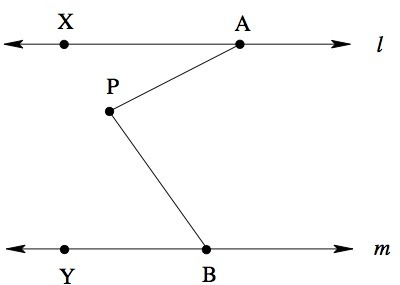# Find the Missing Angle

Alignments to Content Standards: 8.G.A.5

In the picture below, lines $l$ and $m$ are parallel. The measure of angle $\angle PAX$ is $31^\circ$, and the measure of angle $\angle PBY$ is $54^\circ$. What is the measure of angle $\angle APB$?## IM Commentary

This task provides us with the opportunity to see how the mathematical ideas embedded in the standards and clusters mature over time. The task "Uses facts about supplementary, complementary, vertical, and adjacent angles in a multi-step problem to write and solve simple equations for an unknown angle in a figure (7.G.5)" except that it requires students to know, in addition, something about parallel lines, which students will not see until 8th grade. As a result, this task is especially good at illustrating the links between related standards across grade levels.

The Standards for Mathematical Practice focus on the nature of the learning experiences by attending to the thinking processes and habits of mind that students need to develop in order to attain a deep and flexible understanding of mathematics. Certain tasks lend themselves to the demonstration of specific practices by students. The practices that are observable during exploration of a task depend on how instruction unfolds in the classroom. While it is possible that tasks may be connected to several practices, only one practice connection will be discussed in depth. Possible secondary practice connections may be discussed but not in the same degree of detail.

Students who see the hidden structure of the auxiliary line, and make use of that structure by drawing the line (MP. 7), will be able to solve this problem. They can also use the structure of parallel, perpendicular, angles and triangles from earlier experiences to assist them in efficiently solving this multi-step geometric task.  The teacher could use this as a pre-assessment to learn the extent of the student’s understanding of geometric structures and/or as a post-assessment after multiple opportunities to solve similar problems making sure that some of the problems provide opportunities to use variables. Discussion can then be generated based on these various solutions or the understanding of geometric structures that precipitated these solutions.  Ultimately, the discussion should culminate with identifying efficient ways of solving these types of tasks.

## Solution

Extend line AP to intersect line $m$ at point Z.

Since $m$ and $\ell$ are parallel, the alternate interior angles $ZAX$ and $AZB$ have the same measure $31^\circ$.

Angle $PBZ$ (which is the same angle as $PBY$) is given to have measure $54^\circ$.

Since the sum of the angles of a triangle is $180^\circ$ and the sum of $AZB$ and $PBZ$ is $31^\circ + 54^\circ = 85^\circ$, angle $ZPB$ measures $180^\circ - 85^\circ = 95^\circ$.

$ZPB$ and $APB$ together form a straight angle, so their sum is $180^\circ$. So $APB$ measures $180^\circ - 95^\circ = 85^\circ$.

One could also extend $BP$ to meet $\ell$ and proceed similarly.